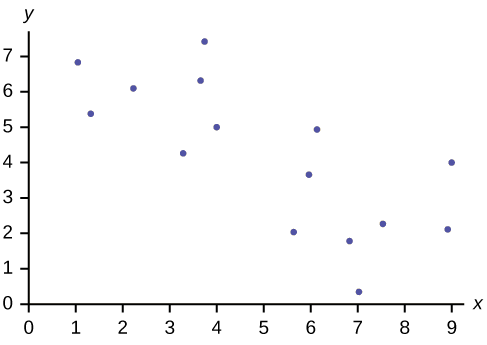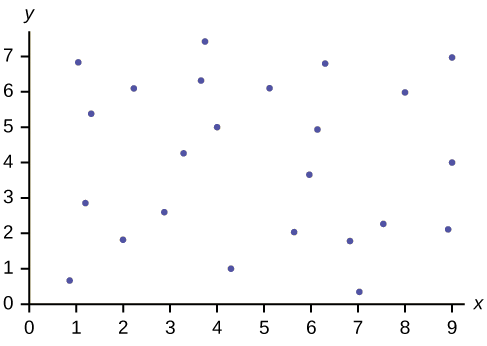# Scatter Plots (Exercises)

Exercise $$\PageIndex{1}$$

Does the scatter plot appear linear? Strong or weak? Positive or negative?Figure $$\PageIndex{4}$$

The data appear to be linear with a strong, positive correlation.

Exercise 12.3.3

Does the scatter plot appear linear? Strong or weak? Positive or negative?Figure $$\PageIndex{5}$$

Exercise 12.3.4

Does the scatter plot appear linear? Strong or weak? Positive or negative?Figure $$\PageIndex{6}$$